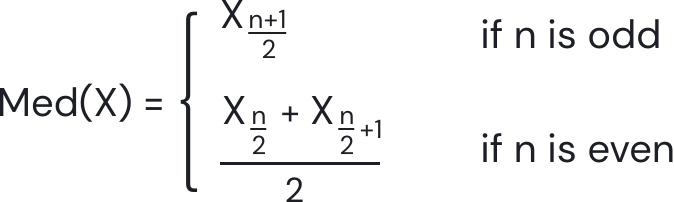### Still have math questions?

Scientific Notation Length Conversion Area Conversion

### Calculator

Mean =
Mode =
Median =
Range =

#### Knowledge

##### Mean

The mean of a list of numbers, is the sum of all of the numbers divided by the number of numbers. Similarly, the mean of a sample X1, X2,...,Xn. Usually written as the sum of the sampled values divided by the number of items in the sample.

m = sum of the terms number of terms

##### Median

The median of a finite list of numbers is the "middle" number, when those numbers are listed in order from smallest to greatest.

If the data set has an odd number of observations, the middle one is selected.

If the data set has an even number of observations. In this case, we find the two numbers in the middle. Then calculate the half value between them.##### Mode

The mode is the value that appears most often in a set of data values. It is a way of expressing, in a (usually) single number, important information about a random variable or a population.

Estimated Mode = L + (fm-fm-1) (fm-fm-1)+(fm-fm+1) × W

L = the lower class boundary of the modal group
fm-1 = the frequency of the group before the modal group
fm = the frequency of the modal group
fm+1 = the frequency of the group after the modal group
w = the group width
Range

The range of a set of data is the difference between the largest and smallest values.

Range = Maximum value - Minimum value

#### Example

What is the mean, median, mode and range:

10, 2, 38, 23, 38, 23, 21

##### Mean

10+2+38+23+38+23+21 7 = 155 7 = 22.14

##### Median

2, 10, 21, 23, 23, 38, 38

##### Mode

2, 10, 21, 23, 23, 38, 38

##### Range

38 - 2 = 36

Please provide numbers only. Please provide at least two numbers. Please provide 2 to 10 non-zero integer numbers. None